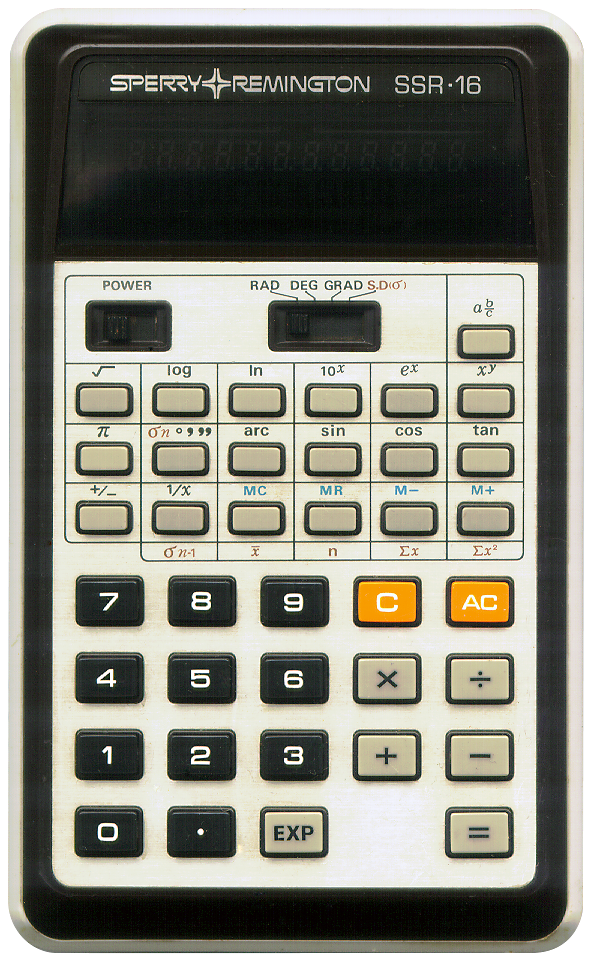# SPERRY REMINGTON SSR-16

## Description

 Brand: SPERRY REMINGTON Model: SSR-16 Type: Scientific calculator Picture:Batteries: AA x 4 Lifetime: Introduced: ca. 1976 Terminated: unknown Notes: A scientific calculator with a fluorescent display, and a slider to switch between modes. This calculator looks very much almost exactly like the Casio fx-19. Not exactly because the Casio fx-19 has 8-digit precision whilst this one has 10 digit precision. I do not know if CASIO also had a 10-digit version that this might be a clone of. The workings of the 'xy' key might be puzzling to users of this calculator. When you enter a (positive) number and press the 'xy' key an intermediate result is shown, being the natural log 'ln' of the number entered. To obtain its power enter the value for y and press the '=' key. My guess is that this calculator uses the formula xy = e(y · ln x). This works nicely for positive values of x but will result in an error when x is zero or less. Not a big problem because powers of negavive numbers behave counter intuitive anyway (producing non-complex results only for integer values of y or fractional values of y with an odd denominator). This calculator has separate keys for the exponent and the constant π instead of the combined key of most other scientific calculators by CASIO (where the "EXP"-key will enable you to enter the numbers exponent when you first enter digits, and will otherwise enter the constant π). There's also no "INV"-key, only an "arc"-key for the inverse trigonometric functions. Statistical functions are available when the mode selector slider is all the way to the right.